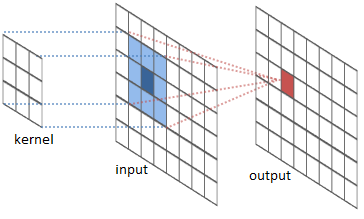# How does training a ConvNet with huge number of parameters on a smaller number of images work?

I have two questions:

1. I am wondering why is that a very deep model such as VGG-16 which has approximately 138 million parameters (Source) can be used as a model to be trained on just 1.3 million images? Even though the authors of VGG-16 used dropout and regularisation to avoid overfitting (source), I just feel that the amount of parameters is still significantly more than the number of images.

2. Is it also true that when you use $$N$$ number of epochs, you "effectively" have $$K*N$$ training datasets, where $$K$$ is the number of training datasets you have? In the case above, $$K$$ = 1.3 million. Personally I don't think this is the case, but I am not sure.

I probably have not done research or read thoroughly, so I would like to apologize if the answers are already somewhere out there. Thank you.

First of all, you are ignoring the dimensionality of the problem. Images are very high-dimensional. Let's say an image has a resolution of $$256 \times 256$$, which means each image has $$65,536$$ pixels. ImageNet images are RGB, so each image has 3 channels, resulting in $$196,608$$ pixels per image. Now, the whole dataset ($$1.3$$m images) has more than $$255$$ billion pixels associated with its images.

Because filters work at pixel-level, the information it gains from one image is more than what a regular ML algorithm would gain from a single training example.As you can see, the kernel (whose weights you want to update, and accounts to 9 parameters in this case) is much smaller than the input image. It would be a mistake to consider a single image (of around $$200,000$$ pixels as we say previously) as only capable of updating one parameter.

Secondly, the input of each layer changes from layer to layer, because of the sequential nature of the network. The second layer sees the output of the first,and so on... This means that the second layer's filters won't see the same image as the first and in fact, as training progresses, their input will also gradually change (because the first layer's filters will be getting more effective).

By the time it reaches let's say the $$M^{th}$$ layer, the input will have changed a lot from the original image, so it would be a mistake to consider that the original image is updating the totality of the parameters in the network.

Thirdly, data augmentation is also a thing. By making simple random transformations to each image (flips, shifts, scales, rotations, brightness/contast adjustments etc.) the network is tricked into thinking that this is a totally new image. This can exponentially increase the size of the dataset.

Finally, you should take a look at where the parameters in a network are. The VGG19 architecture does have more than $$143$$ million, but around $$124$$m of those are from the last 3 FC layers. In fact the first of the three layers has around $$103$$m parameters on its own! This is a very inefficient network design and that the research community has strayed far from recently. A more representative network you should look at is the ResNet architecture. For example a 50-layer ResNet (much deeper than the 19-layered VGG) consists of just around $$25$$m parameters, while achieving a similar -if not better- performance.

• Hi @Djib2011, thanks for your reply. I agree with your first two points that we have billion of pixels. However, the image will still have 4096 inputs (each will be a function of the weights in all the previous convolutional layers) on the first FC layer. Going towards the second FC, which has 4096 hidden units, the number of parameters needed to connect the two FCs is 4096*4097. Do pixels still play some roles here? Oct 4, 2018 at 10:07
• Each one of the connections connects a single parameter to another (the equivalent of a pixel). I mentioned the 4100 neurons in a layer to note how inefficient it is to have so many parameters in a single layer. For example if you drop this first 102m parameter layer completely, how much worse would your model perform? Not much. So by sacrificing a bit of performance you would be reducing the parameters by more than 70%. What I want to say is that probably most of these $4100^2$ connections are pointless (don't impact performance). So it's not fair to make your point in such a network. Oct 4, 2018 at 15:35
• But your point is correct in that this layer much more capacity than necessary and might even be worse, as it might lead your model to overfit more easily. So yes, that many parameters is bad. One final thing you should consider is that FC layers aren't even meant for feature extraction but for classification. So it could be an even simpler model. Anyway, to conclude: yes, the VGG has many more parameters than necessary (evident by other competing CNNs) but no, it isn't correct to compare number of images to number of parameters. Oct 4, 2018 at 15:39